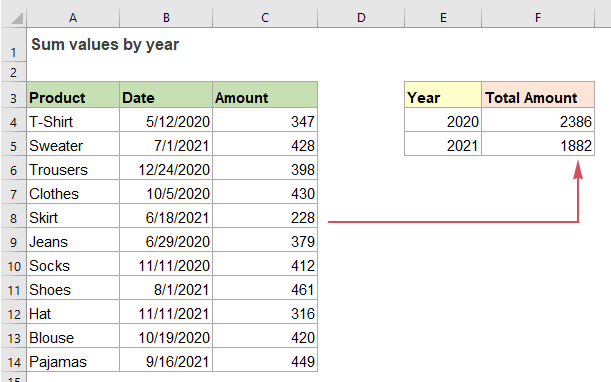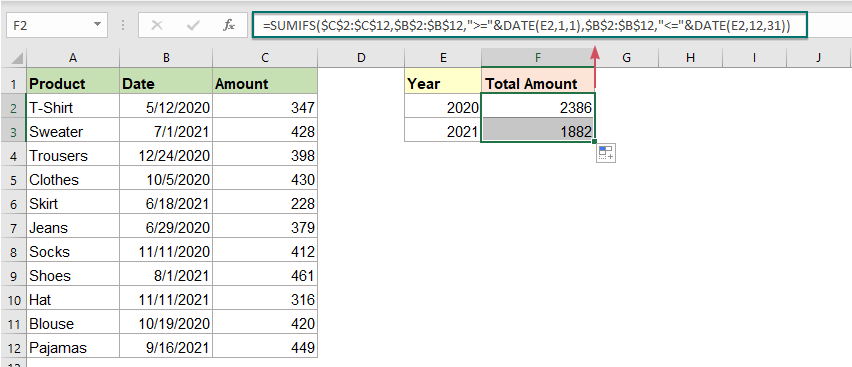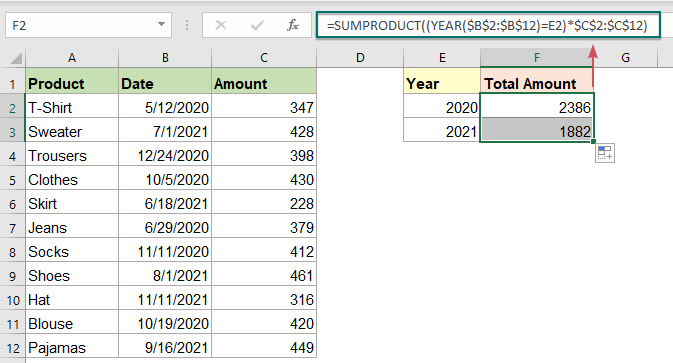## Sum values by year in Excel

Supposing, you have a range of data which contains a list of dates and sale amounts, now you want to get the total amount based on given years as below screenshot shown. In Excel, the normal SUMIFS and SUMPRODUCT functions can help to solve this task quickly and easily. This tutorial will demonstrate how to use these two functions in details.#### Sum values by year in Excel with SUMIFS and DATE functions

To sum total value based on a given year, you should specify the first and last date of the year by using the DATE function, and then use the SUMIFS function to sum all values within the specified dates, the generic syntax is:

=SUMIFS(sum_range,date_range,">="&DATE(year,1,1),date_range,"<="&DATE(year,12,31)
• sum_range: The range of values that to be summed;
• date_range: The range of dates to be tested for the specific year;
• year: The specific year you want to sum values based on. (year, 1,1 indicates the first day of the year, and year,12,31 indicates the last day of the year.)

To solve this task in Excel, please copy or enter the below formula into a blank cell, and then drag down the formula to other cells to get the result:

=SUMIFS(\$C\$2:\$C\$12,\$B\$2:\$B\$12,">="&DATE(E2,1,1),\$B\$2:\$B\$12,"<="&DATE(E2,12,31))Explanation of this formula:

=SUMIFS(\$C\$2:\$C\$12,\$B\$2:\$B\$12,">="&DATE(E2,1,1),\$B\$2:\$B\$12,"<="&DATE(E2,12,31))

• \$C\$2:\$C\$12:is the “sum_range” that contains the values we want to sum.
• \$B\$2:\$B\$12,">="&DATE(E2,1,1): checks for the dates in range B2:B12 that are greater than or equal to 01/01/2020 – the first day of the year in cell E2.
• \$B\$2:\$B\$12,"<="&DATE(E2,12,31): checks for the dates in the range B2:B12 that are less than or equal to 12/31/2020 – the last day of the year in cell E2.
• SUMIFS(\$C\$2:\$C\$12,\$B\$2:\$B\$12,">="&DATE(E2,1,1),\$B\$2:\$B\$12,"<="&DATE(E2,12,31)):After meeting the criteria, the values in the range C2:C12 will be found and summed.

#### Sum values by year in Excel with SUMPRODUCT function

Here, another simple formula which is created by SUMPRODUCT function also can help you, the generic syntax is:

=SUMPRODUCT((YEAR(date_range)=year)*sum_range)
• date_range: The range of dates to be tested for the specific year;
• year: The specific year you want to sum values based on;
• sum_range: The range of values to be summed.

Please copy or enter the following formula into a blank cell, and then drag down the formula to other cells to get the result:Explanation of this formula:

=SUMPRODUCT((YEAR(\$B\$2:\$B\$12)=E2)*\$C\$2:\$C\$12)

• YEAR(\$B\$2:\$B\$12)=E2:The YEAR function returns the year number from each date cell in range B2:B12. This expression checks if the year in E2 equals the year in range B2:B12. And you will get an array like this: {TRUE;FALSE;TRUE;TRUE;FALSE;TRUE;TRUE;FALSE;FALSE;TRUE;FALSE}.
• (YEAR(\$B\$2:\$B\$12)=E2)*\$C\$2:\$C\$12={TRUE;FALSE;TRUE;TRUE;FALSE;TRUE;TRUE;FALSE;FALSE;TRUE;FALSE}*\$C\$2:\$C\$12: In this expression, the TRUEs and FALSEs will be forced to 1s and 0s, and this array is multiplied by each amount in range C2:C12, and you will get a new array like this: {347;0;398;430;0;379;412;0;0;420;0} .
• SUMPRODUCT((YEAR(\$B\$2:\$B\$12)=E2)*\$C\$2:\$C\$12)= SUMPRODUCT({347;0;398;430;0;379;412;0;0;420;0}): The SUMPRODUCT function finally adds up all numbers in this array and returns the result as 2386.

#### Relative function used:

• SUMIFS:
• The SUMIFS function in Excel can help to sum cell values based on multiple criteria.
• SUMPRODUCT:
• The SUMPRODUCT function can be used to multiply two or more columns or arrays together, and then get the sum of products.
• DATE:
• The DATE function can help us to combine year, month and day numbers from separate cells to a valid date.
• YEAR:
• The YEAR function returns the year based on the given date in a 4-digit serial number format.

#### More articles:

• Sum Values By Weeknum Or Weekday
• This tutorial demonstrates formulas step-by-step to help you sum values in a certain range based on the specified week number or weekday in Excel.

### The Best Office Productivity Tools

#### Kutools for Excel - Helps You To Stand Out From Crowd

 Popular Features: Find/Highlight/Identify Duplicates  |  Delete Blank Rows  |  Combine Columns or Cells without Losing Data  |  Round without Formula ... Super VLookup: Multiple Criteria  |  Multiple Value   |  Across Multi-Sheets  |  Fuzzy Lookup... Adv. Drop-down List: Easy Drop Down List  |  Dependent Drop Down List  |  Multi-select Drop Down List... Column Manager: Add a Specific Number of Columns  |  Move Columns  |  Unhide Columns  |  Compare Columns to Select Same & Different Cells ... Featured Features: Grid Focus  |  Design View  |  Big Formula Bar  |  Workbook & Sheet Manager  |  Resource Library (Auto Text)  |  Date Picker  |  Combine Worksheets  |  Encrypt/Decrypt Cells  |  Send Emails by List  |  Super Filter  |  Special Filter (filter bold/italic/strikethrough...) ... Top 15 Toolsets:  12 Text Tools (Add Text, Remove Characters ...)  |  50+ Chart Types (Gantt Chart ...)  |  40+ Practical Formulas (Calculate age based on birthday ...)  |  19 Insertion Tools (Insert QR Code, Insert Picture from Path ...)  |  12 Conversion Tools (Numbers to Words, Currency Conversion ...)  |  7 Merge & Split Tools (Advanced Combine Rows, Split Cells ...)  |  ... and more

Kutools for Excel Boasts Over 300 Features, Ensuring That What You Need is Just A Click Away...

Supports Office/Excel 2007-2021 and 365   |   Available in 44 Languages   |   Easy to Uninstall Completely#### Office Tab - Enable Tabbed Reading and Editing in Microsoft Office (include Excel)

• One second to switch between dozens of open documents!
• Reduce hundreds of mouse clicks for you every day, say goodbye to mouse hand.
• Increases your productivity by 50% when viewing and editing multiple documents.
• Brings Efficient Tabs to Office (include Excel), Just Like Chrome, Edge and Firefox.